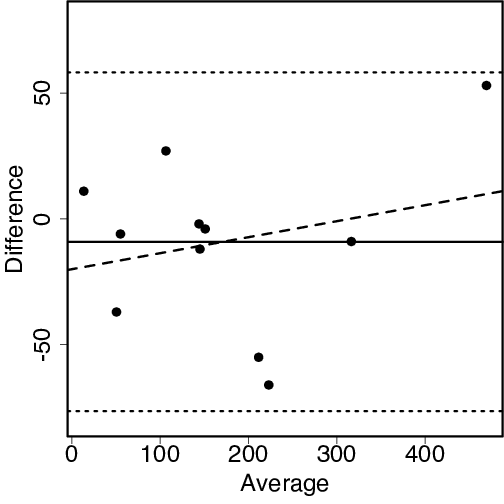## R code used to produce the graph shown below.

``````
hplc <- c(139, 120, 143, 496, 149, 52, 184, 190, 32, 312, 19)
gcms <- c(151, 93, 145, 443, 153, 58, 239, 256, 69, 321, 8)
average <- (hplc + gcms)/2           # Compute average
dif <- (hplc - gcms)                 # and difference
plot(average, dif, ylim=c(-80,80),   # Plot diff vs average
xlab="Average", ylab="Difference", cex=mycex)
limit <- qnorm(.975)
# Add average bias and 95% limits of agreement
abline(h=mean(dif)+c(-limit,0,limit)*sd(dif),lty=c(3,1,3))
# Add line showing bias as function of magnitude
abline(lm(dif~average), lty=2)

``````